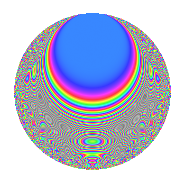# Properties

 Label 630.2.blLevel 630 Weight 2 Character orbit bl Rep. character $$\chi_{630}(41,\cdot)$$ Character field $$\Q(\zeta_{6})$$ Dimension 64 Newform subspaces 2 Sturm bound 288 Trace bound 3

# Related objects

## Defining parameters

 Level: $$N$$ = $$630 = 2 \cdot 3^{2} \cdot 5 \cdot 7$$ Weight: $$k$$ = $$2$$ Character orbit: $$[\chi]$$ = 630.bl (of order $$6$$ and degree $$2$$) Character conductor: $$\operatorname{cond}(\chi)$$ = $$63$$ Character field: $$\Q(\zeta_{6})$$ Newform subspaces: $$2$$ Sturm bound: $$288$$ Trace bound: $$3$$ Distinguishing $$T_p$$: $$13$$

## Dimensions

The following table gives the dimensions of various subspaces of $$M_{2}(630, [\chi])$$.

Total New Old
Modular forms 304 64 240
Cusp forms 272 64 208
Eisenstein series 32 0 32

## Trace form

 $$64q + 32q^{4} - 4q^{7} - 12q^{9} + O(q^{10})$$ $$64q + 32q^{4} - 4q^{7} - 12q^{9} + 12q^{11} + 6q^{14} + 4q^{15} - 32q^{16} + 16q^{18} - 8q^{21} + 72q^{23} - 32q^{25} - 8q^{28} + 12q^{29} + 4q^{30} - 12q^{36} + 16q^{37} + 12q^{39} + 4q^{42} - 8q^{43} - 24q^{46} + 10q^{49} - 40q^{51} + 6q^{56} - 72q^{57} - 4q^{60} + 16q^{63} - 64q^{64} + 12q^{65} + 56q^{67} - 6q^{70} - 16q^{72} - 72q^{74} - 12q^{77} + 64q^{78} + 20q^{79} - 20q^{81} - 22q^{84} + 12q^{85} + 48q^{86} - 48q^{91} + 72q^{92} + 8q^{93} + 36q^{99} + O(q^{100})$$

## Decomposition of $$S_{2}^{\mathrm{new}}(630, [\chi])$$ into newform subspaces

Label Dim. $$A$$ Field CM Traces $q$-expansion
$$a_2$$ $$a_3$$ $$a_5$$ $$a_7$$
630.2.bl.a $$32$$ $$5.031$$ None $$0$$ $$-4$$ $$-16$$ $$-2$$
630.2.bl.b $$32$$ $$5.031$$ None $$0$$ $$4$$ $$16$$ $$-2$$

## Decomposition of $$S_{2}^{\mathrm{old}}(630, [\chi])$$ into lower level spaces

$$S_{2}^{\mathrm{old}}(630, [\chi]) \cong$$ $$S_{2}^{\mathrm{new}}(63, [\chi])$$$$^{\oplus 4}$$$$\oplus$$$$S_{2}^{\mathrm{new}}(126, [\chi])$$$$^{\oplus 2}$$$$\oplus$$$$S_{2}^{\mathrm{new}}(315, [\chi])$$$$^{\oplus 2}$$

## Hecke Characteristic Polynomials

There are no characteristic polynomials of Hecke operators in the database